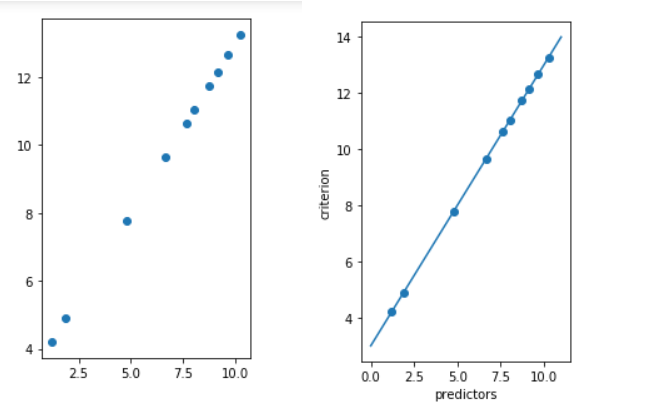Open in App
Not now

# ML | Types of Regression Techniques

• Difficulty Level : Medium
• Last Updated : 25 Jan, 2023

When Regression is chosen?
A regression problem is when the output variable is a real or continuous value, such as “salary” or “weight”. Many different models can be used, the simplest is linear regression. It tries to fit data with the best hyperplane which goes through the points.

### Introduction:

Regression analysis is a statistical technique used to model and understand the relationship between a dependent variable and one or more independent variables. There are several types of regression techniques, each suited for different types of data and different types of relationships. The main types of regression techniques are:

Linear Regression: This is the most basic form of regression analysis and is used to model a linear relationship between a single dependent variable and one or more independent variables.

Polynomial Regression: This is an extension of linear regression and is used to model a non-linear relationship between the dependent variable and independent variables.

Logistic Regression: This is used for classification tasks, where the goal is to predict a binary outcome (e.g., yes/no, true/false) based on one or more independent variables.

Decision Tree Regression: This is a non-parametric method used to model a decision tree to predict a continuous outcome.

Random Forest Regression: This is an ensemble method that combines multiple decision trees to improve the predictive performance of the model.

Support Vector Regression (SVR): This is a linear model for regression tasks, it can also handle non-linear relationships by mapping the data into a higher dimensional space.

Ridge Regression: This is a regularized linear regression model, it tries to reduce the model complexity by adding a penalty term to the cost function.

Lasso Regression: This is another regularized linear regression model, it works by adding a penalty term to the cost function, but it tends to zero out some features’ coefficients, which makes it useful for feature selection.

Neural Network Regression: This is a non-linear model that uses a neural network to model the relationship between the independent and dependent variables.

Regression Analysis is a statistical process for estimating the relationships between the dependent variables or criterion variables and one or more independent variables or predictors. Regression analysis explains the changes in criteria in relation to changes in select predictors. The conditional expectation of the criteria is based on predictors where the average value of the dependent variables is given when the independent variables are changed. Three major uses for regression analysis are determining the strength of predictors, forecasting an effect, and trend forecasting.

Types of Regression:

• Linear regression is used for predictive analysis. Linear regression is a linear approach for modelling the relationship between the criterion or the scalar response and the multiple predictors or explanatory variables. Linear regression focuses on the conditional probability distribution of the response given the values of the predictors. For linear regression, there is a danger of overfitting. The formula for linear regression is: Y’ = bX + A.
• Polynomial regression is used for curvilinear data. Polynomial regression is fit with the method of least squares. The goal of regression analysis is to model the expected value of a dependent variable y in regards to the independent variable x. The equation for polynomial regression is: l =• Stepwise regression is used for fitting regression models with predictive models. It is carried out automatically. With each step, the variable is added or subtracted from the set of explanatory variables. The approaches for stepwise regression are forward selection, backward elimination, and bidirectional elimination. The formula for stepwise regression is• Ridge regression is a technique for analyzing multiple regression data. When multicollinearity occurs, least squares estimates are unbiased. A degree of bias is added to the regression estimates, and as a result, ridge regression reduces the standard errors. The formula for ridge regression is• Lasso regression is a regression analysis method that performs both variable selection and regularization. Lasso regression uses soft thresholding. Lasso regression selects only a subset of the provided covariates for use in the final model. Lasso regression is.
• ElasticNet regression is a regularized regression method that linearly combines the penalties of the lasso and ridge methods. ElasticNet regression is used for support vector machines, metric learning, and portfolio optimization. The penalty function is given by:.
Below is the simple implementation:

## Python3

 # importing libraries import numpy as np import matplotlib.pyplot as plt from sklearn.linear_model import LinearRegression   x = 11 * np.random.random((10, 1))   # y = a * x + b y = 1.0 * x + 3.0   # create a linear regression model model = LinearRegression() model.fit(x, y)   # predict y from the data where the x is predicted from the x x_pred = np.linspace(0, 11, 100) y_pred = model.predict(x_pred[:, np.newaxis])   # plot the results plt.figure(figsize =(3, 5)) ax = plt.axes() ax.scatter(x, y)   ax.plot(x_pred, y_pred) ax.set_xlabel('predictors') ax.set_ylabel('criterion') ax.axis('tight')   plt.show()

Output:My Personal Notes arrow_drop_up
Related Articles